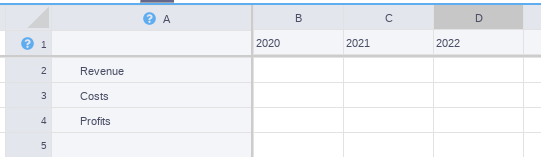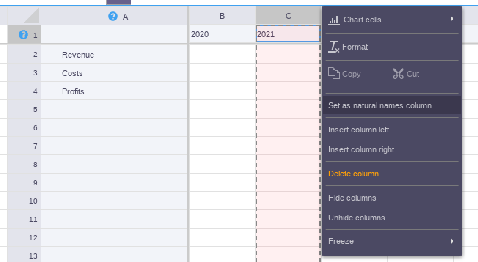# Import from Excel

Contents

If your file does not have the characteristics listed below, and it cannot be modified to be imported successfully, you can get our Excel Add-in and keep working in your Excel file (some Visyond features will not be available in the add-in).

#### Format of the Excel file

Your file should be in .xls or .xlsx format.

Should not contain more than:

• 10,000 rows
• 1,000 columns
• 150 worksheets.

Should not contain:

• Macros and formulas linking to them: our team can transform them into custom modules if needed;
• Formulas with ranges in square brackets ( e.g., ‘=SUM([A1]), or ‘=SUM([this_is_a_named_range]) ): use explicit ranges instead (e.g., ‘=SUM(A1)’); ;
• Array Constants (e.g., ‘=SUM(A1:E1*{1,2,3,4,5}’)
• Structured references to Excel tables (e.g., ‘=SUM(DeptSales[Sales Amount]’ ): use explicit ranges instead (e.g., ‘=SUM(C2:C7)’);
• DATABASE functions and queries;
• @ and # symbols;

If the file contains links to other Excel files, you will find the names of the external files in the External Sources Panel and will have to upload those files too.

#### Prepare the file to extract human-readable names

Visyond uses human-readable names so you can type formula either as =A1-B1 or as  =Revenues-Costs. See How to use Natural Names in Visyond.

Once you have imported the spreadsheet, you will see Row 1 and Column A as the default data providers for natural names (they have a different background color).At any point you can change the row and/or column used as data provider by right-clicking on them and clicking on “Set as natural name” option in the context menu.#### Import time

Importing may take some time as we need to extract metadata to create Visyond data structures (richer than traditional spreadsheets).

You can close the import window and work on other projects while we are importing. We will send you an email when the project is ready.

#### Array Formulas

Excel syntax for array formulas uses curly braces by pressing Control + Shift + Enter:

{=SUM(SQRT(…)}

Visyond achieves the same result by using the ARRAYFROMULA function:

=ARRAYFORMULA(SUM(SQRT(…)))

Visyond can also use the ARRAY_CONSTRAIN function to clip the expansion of the array to a specific number of rows and columns. For example:

=ARRAY_CONSTRAIN(ARRAYFORMULA(SUM(SQRT())), 2, 3)

This will expand the formula in a 2 rows by 3 columns range.

#### The Import Report

We compare the import results with the original Excel file and prepare a report with the unsupported or unrecognised elements so you can easily identify them and decide what to do. The import report includes:

• List of cells that have different values in Excel and Visyond; this can be due to:
• Differences in floating point precision, usually negligible differences at the 7th decimal digit
• Local dates ambiguities (e.g., is “11/12/2012” December 11th or November 12th ?)
• Other local conventions (e.g., decimal digits vs thousands separators).
• List of cells with unsupported functions (wrapped in Visyond’s IMPORT_ERROR function). We do not support:
• Some type of named ranges
• Array Functions

We do not lose the Excel value and formulae for the unsupported functions: they are wrapped in Visyond’s IMPORT_ERROR function and the formula in the cell looks like this:

=IMPORT_ERROR(“Excel_formula_containing_usopported_function”, EXCEL_VALUE)

This way you can identify the cell and decide what to do. Meanwhile, the Excel value is reused in the calculations.